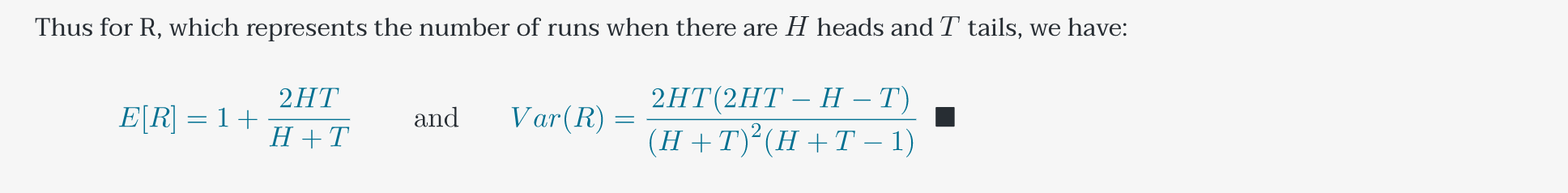# Probability: Finding The Expectation and Variance of Runs

Last week, I completed a summer course at Stanford: CS109, Probability for Computer Scientists. This 8-week long class was intense and challenging but one of the most rewarding classes I've taken at Stanford so far. A few weeks ago, I had an idea for a homework problem but I couldn't quite figure out how to implement the idea. The problem is the following: Below are two sequences of 300 “coin flips” (H for heads, T for tails). One of these is a true sequence of 300 independent flips of a fair coin. The other was generated by a person typing out H’s and T’s and trying to seem random. Which sequence is the true sequence of coin flips? Make an argument that is justified with probabilities calculated on the sequences. Both sequences have 148 heads, two less than the expected number for a 0.5 probability of heads.